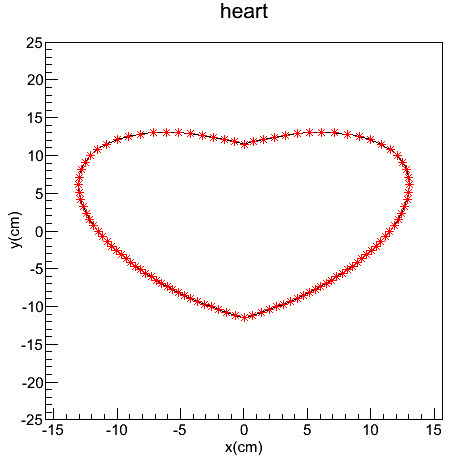# SetRangeUser doesn't work well

I want to use SetRangeUser to limit the range of axis, however, I got a very strange result.
It works fine for y axis, but not for x axis.

Assuming the range x of my graph is -15 cm to 15 cm, and now if

axis->SetRangeUser(-25.0, 25.0); //doesn’t work at all

else if

axis->SetRangeUser(-10.0, 25.0); //works fine for left part

else if

axis->SetRangeUser(-25.0, 10.0); //works fine for right part

else if

axis->SetRangeUser(-10.0, 10.0); //works fine for double sides

Below is the code I used.

Thanks a lot.

[code] const Int_t n = 100;//number of points

Double_t x[n], y[n];//array to store coordinates of points
Double_t xx[n], yy[n];//array to store coordinates of points

Double_t theta, rho; //polar coordinate, angle and radius

const Double_t cm = 10.0;
for(Int_t i = 0; i!=n; i++)
{
theta = i2TMath::Pi()/n;
//here I use 2250 to make x and y range is [-15 cm, 15 cm]
rho = std::sqrt(2250cmcm/(17-16*sin(theta)*sqrt( cos(theta)*cos(theta) ) ) );

``````x[i] = rho*cos(theta);
y[i] = rho*sin(theta);

xx[i] = x[i]/cm;
yy[i] = y[i]/cm;
``````

// std::cout<<"(x,y)_"<< i <<": " <<"(" << x[i]/cm <<","<<y[i]/cm << “) cm”<<std::endl;

}

TCanvas* c1 = new TCanvas(“c1”, “a heart”, 200, 200, 500, 500);

// TGraph* graph01 = new TGraph(n,x,y);
TGraph* graph01 = new TGraph(n,xx,yy);//for the plot, the unit on each axis is cm

graph01->SetTitle(“heart”);

TAxis* axis = graph01->GetXaxis();
axis->SetTitle(“x(cm)”);
axis->CenterTitle(kTRUE);
axis->SetTitleOffset(1.1);
axis->SetRangeUser(-25.0, 25.0);

axis = graph01->GetYaxis();
axis->SetTitle(“y(cm)”);
axis->CenterTitle(kTRUE);
axis->SetTitleOffset(1.1);
axis->SetRangeUser(-25.0, 25.0);

graph01->SetMarkerColor(2);
graph01->SetMarkerStyle(1);
graph01->SetLineWidth(10);
graph01->SetLineColor(2);

graph01->Draw(“AC*”);[/code]Try:
MyGraph->GetXaxis()->SetLimits(xmin, xmax);
MyGraph->GetYaxis()->SetRangeUser(ymin, ymax);
or:
MyGraph->GetXaxis()->SetLimits(xmin, xmax);
MyGraph->GetHistogram()->SetMinimum(ymin);
MyGraph->GetHistogram()->SetMaximum(ymax);